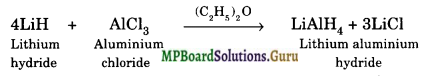# MP Board Class 11th Chemistry Solutions Chapter 11 The p-Block Elements

In this article, we will share MP Board Class 11th Chemistry Solutions  Chapter 11 The p-Block Elements Pdf, These solutions are solved by subject experts from the latest edition books.

## MP Board Class 11th Chemistry Solutions Chapter 11 The p-Block Elements

### MP Board Class 11 Chemistry The p-Block Elements Textbook Questions and Answers

Question 1.
Discuss the pattern of variation in the oxidation states of
(i) B to Tl
(ii) C to Pb.
Solution.
(i) The oxidation state in a group depends upon the presence of electrons in valence shell of an element. B and A1 have no d-or f-electrons, therefore, they do not exhibit inert pair effect. In other words, as we move down the group from Ga to Tl, the stability of+1 oxidation state increases (t.e., Ga < In < Tl) while that of +3 oxidation state decreases (i.e., Ga > In > Tl). Thus, + 1 oxidation state of Tl is more stable than its +3 oxidation state.

(ii) Carbon and silicon do not contain d- or f-electrons and hence do not exhibit inert pair effect. In other words, as we move down the group from Ge to Pb, the stability of +2 oxidation state increases (i.e., Ge < Sn < Pb) while that of +4 oxidation state decreases (i.e., Ge > Sn > Pb). Thus, +2 oxidation state of Pb is more stable than its +4 oxidation state.

Question 2.
How can you explain higher stability of BCl3 as compared to TlCl3?
Solution.
Boron and thallium belong to group 13 of the periodic table. On moving from i top to bottom in a group the increase in nuclear charge is not neutralised by the poor shielding effect of electrons in inner orbitals (i.e., 3d, 4d, 5d, 4f, etc). As a result, the inert pair effect becomes more predominant. Thus, the s electrons experience strong ” force of attraction. The inert pair effect is maximum in Tl.

As a result, only 6p1 electrons participate in bond formation and thus the most stable state of Tl is +1 and not +3. Therefore, TlCl is stable but TlCl3 is unstable. In contrast, due to the absence of d- and f-electrons in B, all the three valence electrons (i.e., two 2s and one 2p) take part in bond formation and hence B exhibits an oxidation state of +3 and thus forms BCl3. Thus, BCl3 is more stable than TlCl3.

Question 3.
Why does boron trifluoride behave as a Lewis acid?
Solution.
The B atom in BF3 has only 6 electrons in the valence shell and thus needs two more electrons to complete its octet. Therefore, it easily accepts a pair of electrons.

Question 4.
Consider the compounds, BCl3 and CCl4. How will they behave with water?
Solution.
The B atom in BCl3 has only six electrons in the valence shell and hence is an electron-deficient molecule. It easily accepts. a pair of electrons donated by water and hence BCl3 undergoes hydrolysis to form boric acid (H3BO3) and HCl.
BCl3 + 3H2O → H3BO3 + 3HCl
In contrast, C atom in CCl4 has 8 electrons in the valence shell. Therefore, it is an electron-precise molecule. As a result, it neither accepts nor donates a pair of electrons. In simple words, CCl4 does not undergo hydrolysis in water.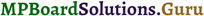Question 5.
Is boric acid a protic acid? Explain.
Solution.
A protic acid is the one which can ionise in solutions to produce protons or hydronium ions. Boric acid is not a protic acid since it does not ionize in H2O to give a proton.
H3BO3 + H2O → H2BO3 + H3O+

Question 6.
Explain what happens when boric acid is heated?
Solution.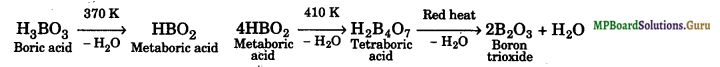Question 7.
Describe the shapes of BF3 and [BF4]. Assign the hybridization of boron in these species.
Solution.
In BF3, boron is sp2-hybridized and, therefore, BF3 is a planar molecule. On the other hand, in [BF4], boron is sp3-hybridized and hence [BF4] is a tetrahedral species.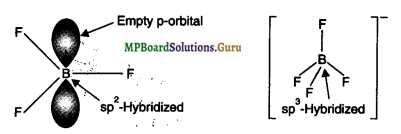Question 8.
Write reactions to justify amphoteric nature of aluminium.
Solution.
An amphoteric substance is the one which can react with both acid as well as base. Aluminium dissolves both in acids and alkalies evolving dihydrogen.
2Al(s) + 3H2SO4(aq) → Al2 (SO4)3 (aq) + 3H2(g)
2Al(s) + 2NaOH(aq) + 6H2O(l) → 2Na+[Al(OH)4](aq) + 3H2(g) Sod. tetrahy droxoaluminate (III)

Question 9.
What are electron deficient compounds? Are BCl3 and SiCl4 electron-deficient species? Explain.
Solution.
Species in which the central atom does not have eight electrons in the valence shell or those which have 8 electrons in the valence shell but can expand their covalency beyond 4 due to the presence of d-orbitals, are called electron deficient molecules.
(i) In BCl3, the central boron atom has only six electrons. Therefore, it is an electron-deficient compound. As such it accepts a pair of electrons from nucleophiles like NH3 to form an adduct.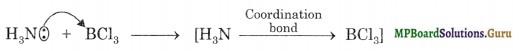(ii) In SiCl4, the central Si atom has 8 electrons but it can expand its covalency beyond 4 due to the presence of vacant d-orbitals. Therefore, in principle, SiCl4 should also be an electron-deficient molecule. It is noteworthy to mention here that SiCl4 cannot accept two more Cl ions to form [SiCl6]2-.

Question 10.
Write the resonance structures of CO32- and HCO3.
Solution.
Resonance structures of CO32- ion: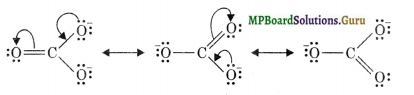Resonance structures of HCO3 ion: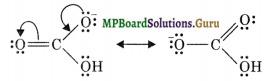Question 11.
What is the state of hybridization of carbon in (a) CO32- (b) diamond (c) graphite?
Solution.
(a) sp2
(b) sp3
(c) sp2

Question 12.
Explain the difference in properties of diamond and graphite on the basis of their structures.
Solution.

 Diamond Graphite 1. It is a transparent substance 1. It is black and opaque 2. Very hard 2. Soft 3. Bad conductor of heat and electricity 3. Good conductor of heat and electricity 4. It possesses three-dimensional network structure 4. It possesses a sheet-type structure 5. Chemical reactivity is low 5. Chemical reactivity is high

Question 13.
Rationalise the given statements and give chemical reactions:
(i) lead (II) chloride reacts with Cl2 to give PbCl4
(ii) lead (IV) chloride is highly unstable towards heat
(iii) lead is known not to form an iodide, PbI4
Solution.
(i) Pb can exist in its compounds in two different oxidation states of+2 and +4.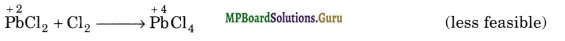Due to inert pair effect, Pb is more stable in + 2 than in + 4 oxidation state. Therefore, lead (ID chloride is more stable than lead (IV) chloride and hence lead (II) chloride does not react with Cl2 to form lead (IV) chloride.

(ii) Lead (IV) chloride on heating decomposes to give lead (II) chloride and Cl2.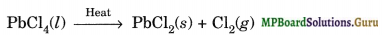PbCl4 is unstable to heat because Pb in + 2 oxidation state is more stable than its + 4 oxidation state due to inert pair effect.

(iii) Lead (IV) Iodide is not formed because the Pb-I bond initially formed during the reaction does not release enough energy to unpair 6s2 electrons and excite one of them to the higher 6p -orbital to have four unpaired electrons around lead atom needed for formation of Pbl4. In addition to this Pb+4 ion acts as a good oxidising agent (i.e readily changes to stable Pb+2 ion) and I- ion has good reducing power. Hence Pbl4 does not exist.Question 14.
Suggest a reason why the B-F bond lengths in BF3(130 pm) and BF4 (143 pm) differ?
Solution.
BF3 is a planar molecule in which B is sp2-hybridized. It has an empty 2p- orbital. F-atom has three lone pairs of electrons in the 2p-orbitals. Because of its small size and strong inter electronic repulsions, pn-pn back bonding or back donation occurs in which a lone pair is transferred from F to B.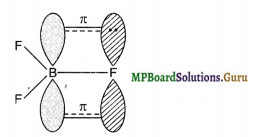As a result of this back bonding, B-F bond acquires some double-bond character. In contrast, in [BF4] ion, B is sp3-hybridized and hence does not have an empty p-orbital available to accept the electrons donated by the F atom. Consequently, in [BF4], B-F is a purely single bond. Since double bonds are shorter than single bonds, therefore, the B-F bond length in BF3 is shorter (130 pm) than B-F bond length (143 pm) in [BF4].

Question 15.
If B-Cl bond has a dipole moment, why does BCl3 have zero dipole moment?
Solution.
B-Cl bond has a dipole moment due to electronegativity difference between B (E.N. = 2.0) and Cl (E.N. = 3.0). The overall dipole moment of a molecule, however, depends upon its geometry. Now BCl3 is a planar molecule in which the three B-Cl bonds are inclined at an angle of 120°. Therefore, the resultant of two B-Cl bonds is cancelled by equal and opposite dipole moment of the third B-Cl bond.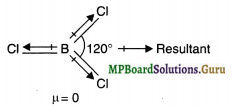As a result, overall dipole moment of BCl3 is zero.

Question 16.
Aluminium trifluoride is insoluble in anhydrous HF but dissolves on addition of NaF. Aluminium trifluoride precipitates out of the resulting solution when gaseous BF3 is bubbled through. Give reasons?
Solution.
(i) Anhydrous HF is a covalent compound and is strongly hydrogen-bonded. Therefore, it does not give free F ions and hence AlF3 does not dissolve in HF. In contrast, NaF is an ionic compound and hence F ions are easily available. As a result these combine with AlF3 to form the soluble complex.
3NaF + AlF3 → Na3 [AlF6] Sod. hexafluoroaluminate (III) (soluble complex)

(ii) On bubbling gaseous BF3, AlF3 is precipitated.
It is because BF3 is a stronger lewis acid than AlF3. This is attributable to smaller size and higher electronegativity of Boron. As a result of this B has a much higher tendency to form complexes than Al, therefore when BF3 is added to the above solution, AlF3 gets precipitated.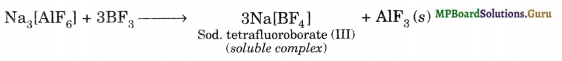Question 17.
Suggest a reason as to why CO is poisonous?
Solution.
As a result, it destroys the oxygen-carrying capacity of haemoglobin and ultimately the man dies of suffociation. The highly poisonous nature of CO arises due to its ability to form carboxyhaemoglobin with haemoglobin which is about 300 times more stable than the oxygen-haemoglobin complex.

Question 18.
How is excessive content of CO2 responsible for global warming?
Solution.
If the concentration of CO2 increases beyond a certain level due to excessive combustion, some of the CO2 will always remain unutilized. This excess CO2 absorbs heat radiated by the earth. Some of it is dissipated into the atmosphere while the remaining part is radiated back to the earth and other bodies present on the earth. As a result, temperature of the earth and other bodies on the earth increases. This is called greenhouse effect and CO2 is called a greenhouse gas.

Question 19.
Explain structures of diborane and boric acid.
Solution.
(i) Structure of Diborane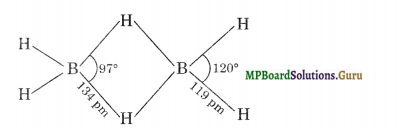In the above structure the four-terminal hydrogen atoms and the two boron atoms lie in one plane. Above and below this plane, there are two bridging hydrogen atoms. The four-terminal B-H 1 aids are regular two-centre – two electron bonds. These bonds are normally covalent bonds and hence are quite strong.
The two bridge bonds B-H-B are quite different from normal covalent bonds. Each bridge hydrogen is bonded to two boron atoms by a pair of electrons. In other words, these are three centre, two-electron bonds, hence these bonds are quite weak. Because of their resemblance to a banana, these three centre bonds are called banana bonds.
(ii) Structure of Boric acid: It contains planar BO33- units which are bonded together through hydrogen bonds forming a trigonal planar layer structure. Hydrogen atoms act as a bridge between two oxygen atoms of different BO33- units.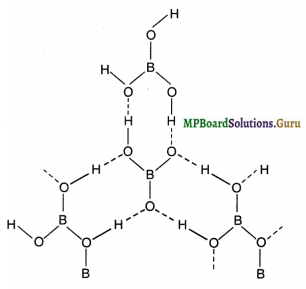Question 20.
What happens when
(a) Borax is heated strongly
(b) Boric acid is added to water
(c) Aluminium is treated with dilute NaOH
(d) BF3 is reacted with ammonia
Solution.
(a) When borax is heated strongly, a transparent glassy bead which consists of sodium metaborate (NaBO2) and boric anhydride is formed.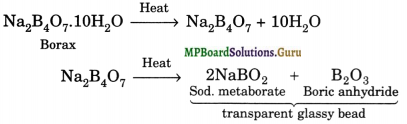(b) Boric acid acts as a weak Lewis acid. When boric acid is added to water it accepts the hydroxyl ion of water and releases a proton into the solution as shown: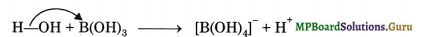(c) Aluminium when treated with dil NaOH dissolves in it forming sodium tetra hydroxoaluminate complex along with evolution of dihydrogen gas.
2Al(s) + 2NaOH(aq) + 6H2O(l) → 2Na+[Al(OH)4](aq) + 3H2(g)
(d) BF3 is a Lewis acid and accepts a pair of electrons from NH3 to form a complex.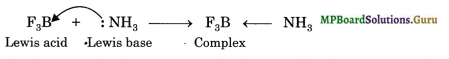Question 21.
Explain the following reactions
(a) Silicon is heated with methyl chloride at high temperature in the presence of copper
(b) Silicon dioxide is treated with hydrogen fluoride
(c) CO is heated with ZnO
(d) Hydrated alumina is treated with aqueous NaOH solution.
Solution.
(a) The reaction of silicon with CH3Cl in the presence of Cu, at high temperature produces a mixture of mono-, di- and trimethylchlorosilane along with a small amount of tetramethylsilane.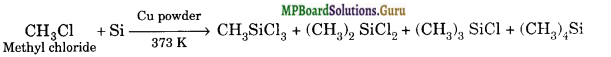(b) Silicon tetrafluoride dissolves in HF to from hydro fluorosilicic acid
SiO2 + 4HF → SiF4 + 2H2OL
SiF4 + 2HF → H2SiF6

(c) ZnO is reduced to zinc metal
ZnO + C → Zn + CO

(d) Alumina dissolves to form sodium meta aluminate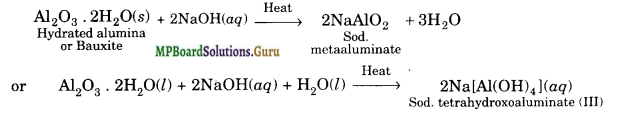Question 22.
Give reasons:
(i) Cone. HNO3 can be transported in an aluminium container.
(ii) A mixture of dilute NaOH and aluminium pieces is used to open drains.
(iii) Graphite is used as a lubricant.
(iv) Diamond is used as an abrasive.
(v) Aluminium alloys are used to make aircraft body.
(vi) Aluminium utensils should not be kept in water overnight.
(vii) Aluminium wire is used to make transmission cables.
Solution.
(i) Al reacts with cone. HNO3 to form a very thin film of aluminium oxide on its surface which protects it from further action.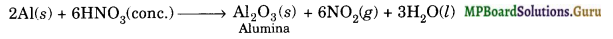Thus, Al becomes passive and hence aluminium containers can be used to transport cone. HNO3.
(ii) NaOH reacts with A1 to evolve dihydrogen gas whose high pressure is enough to open clogged drains.
2Al(s) + 2NaOH(aqr) + 2H2O(l) → 2NaAlO2(aq) + 3H2(g)

(iii) Graphite has a layered structure in which the different layers are held together by weak van der Waal’s forces and hence can be made to slip over one another. Therefore, graphite acts as a lubricant.

(iv) Diamond is very hard because it is a network solid and hence can be used as an abrasive.
(v) Aluminium alloys such as duralumin are light, tough and resistant to corrosion and hence are used to make aircraft body.

(vi) A1 reacts with H20 and dissolved 02 to form a thin film of aluminium oxide.
2Al(s) + O2(g) + H2O (l) → Al2O3(S) + H2(g)
A very very small amount of Al2O3 may also dissolve to give a few ppm of Al3+ ions in the solution. Since Al3+ ions are injurious to health, therefore, drinking water should not be kept in aluminium utensils overnight.

(vii) On weight to weight basis, aluminium conducts electricity twice as Cu. Therefore, it is used in transmission cables.

Question 23.
Explain why there is a phenomenal decrease in ionization enthalpy from carbon to silicon.
Solution.
Due to increase in atomic size and screening effect, the force of attraction of the nucleus for the valence electron decreases considerably in Si as compared to C. As a result, there is a phenomenal decrease in ionization enthalpy from carbon to silicon.

Question .24.
How would you explain the lower atomic radius of Ga as compared to Al?
Solution.
Due to poor shielding of the valence electrons of Ga by the inner 3d- electrons, the effective nuclear charge of Ga is greater in magnitude than that of Al. As a result, the electrons in gallium experience greater force of attraction by the nucleus than in Al and hence atomic size of Ga (135 pm) is slightly less than that of Al (143 pm).

Question 25.
What are allotropes? Sketch the structure of two allotropes of carbon namely diamond and graphite. What is the impact of structure on physical properties of two allotropes?
Solution.
An amphoteric substance is the one which can react with both acid as well as base. Aluminium dissolves both in acids and alkalies evolving dihydrogen.
2Al(s) + 3H2SO4(aq) → Al2 (SO4)3 (aq) + 3H2(g)
2Al(s) + 2NaOH(aq) + 6H2O(l) → 2Na+[Al(OH)4](aq) + 3H2(g) Sod. tetrahy droxoaluminate (III)

Question 26.
Classify following oxides as neutral, acidic, basic or amphoteric
CO, B2O3, SiO2, CO2, Al2O3, PbO2, Tl2O3
Solution.
The given oxides can be classified as:
Neutral oxides: CO ;
Acidic oxides: B2O3, SiO2, CO2
Amphoteric oxides: Al2O3, PbO2 Basic oxide: Tl2O3.Question 27.
In some of the reactions, thallium resembles aluminium, whereas in others it resembles with group I metals. Support this statement by giving some evidences.
Solution.
Aluminium shows a uniform oxidation of+3 in its compounds. Like aluminium, Tl also shows +3 oxidation state is some of its compounds like TiCl3, Tl2O3 etc. Al is known to form octahedral complexes like [AIF6]3-. Similarly, T1 also forms octahedral complexes as [TlF6]3-.
Thallium also resembles group 1 metals. Like group 1 metals which show a stable oxidation state of +1 in their compounds Tl, due to inert pair effect, also shows +1 oxidation state in some of its compounds such as Tl2O, TlCl, TlClO4 etc.
Similarly, like group 1 oxides, Tl2O is strongly basic.

Question 28.
When metal X is treated with sodium hydroxide, a white precipitate (A) is obtained, which is soluble in excess of NaOH to give soluble complex (B). Compound (A) is soluble in dilute HC1 to form compound (C). The compound (A) when heated strongly gives (D), which is used to extract metal. Identify (X), (A), (B), (C) and (D). Write suitable equations to support their identities.
Solution.
Since metal X reacts with NaOH to first give a white ppt. (A) which dissolves in excess of NaOH to give a soluble complex (B), therefore, metal (X) ipust be A1; ppt (A) must be A1(0H)3 and complex (B) must be sodium tetra hydroxoaluminate (III)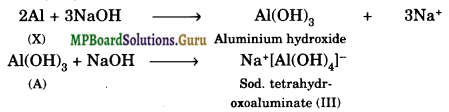Since (A) is amphoteric in nature, it reacts with dil. HCl to form (C) which is AlCl3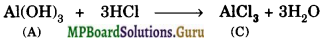Since (A) on heating gives (D) which is used to extract metal, therefore, (D) must be alumina (Al2O3)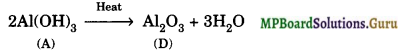Question 29.
What do you understand by (a) inert pair effect (b) allotropy (c) catenation?
Solution.
(a) Inert pair effect: The resistance of a pair of s-electrons to be lose or to participate in covalent bond formation is known as inert pair effect.
(b) Allotropy: It is a phenomenon of existence of different forms of element having different physical properties.
(c) Catenation: This property of forming the chains of identical atoms is called catenation.

Question 30.
A certain salt X, gives the following results.
(i) Its aqueous solution is alkaline to litmus.
(ii) It swells up to a glassy material Y on strong heating.
(iii) When cone. H2SO4 is added to a hot solution of X, white crystals of an acid Z separate out.
Write equations for all the above reactions and identify X, Y and Z.
The following inferences can be drawn from the given results:
Solution.
(i) As the aqueous solution of salt (X) is alkaline to litmus, it must be the salt of a strong base and a weak acid.

(ii) Since the salt (X) swells up to a glassy material (Y) on strong heating, therefore, (X) must be borax and (Y) must be a mixture of sodium metaborate and boric anhydride.

(iii) When cone. H2SO4 is added to a hot solution of X, i.e., borax, white crystals of an acid (Z) separate out, therefore, (Z) must be orthoboric acid. The equations for the reac¬tions involved in the question are:
Watch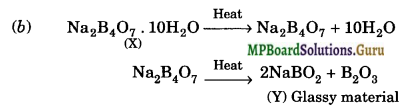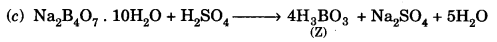Question 31.
Write balanced equations for:
(i) BF3 + LiH →
(ii) B2H6 + H2O →
(iii) NaH + B2H6
(iv) img
(v) Al + NaOH →
(vi) B2H6 + NH3
Solution.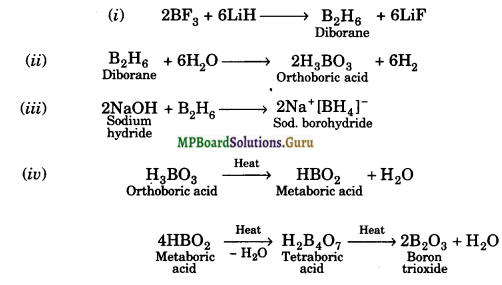(v) 2Al + 2NaOH + 6H2O → 2Na[Al(OH)4] + 3H2
(vi) B2H6 + 2NH3 → 2BH3 . NH3.

Question 32.
Give one method for industrial preparation and one for laboratory preparation of CO and CO2 each.
Solution.
The methods of preparation of CO and CO2 are :
(a) Carbon monoxide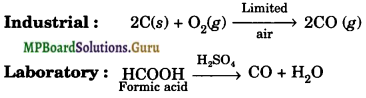(b) Carbon dioxide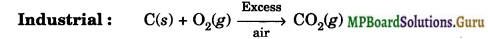Laboratory : CaCO3(s) + 2HCl(aq) → CaCl2 (aq) + CO2(g) + H2O(l)

Question 33.
An aqueous solution of borax is :
(a) neutral
(b) amphoteric
(c) basic
(d) acidic
Solution.
Borax is a salt of a strong base (NaOH) and a weak acid (H3BO3), therefore, it is basic in nature, i.e., option (c) is correct.

Question 34.
Boric acid is polymeric due to:
(a) its acidic nature
(b) the presence of hydrogen bonds
(c) its monobasic nature
(d) its geometry
Solution.
Boric acid is polymeric due to the presence of H-bonds. Therefore, option (b) is correct.

Question 35.
The type of hybridisation of boron in diborane is:
(a) sp
(b) sp2
(c) sp3
(d) dsp2
Solution.
In B2H6, B is sp3-hybridized. Therefore, option (c) is correct.

Question 36.
Thermodynamically the most stable form of carbon is:
(a) diamond
(b) graphite
(c) fullerenes
(d) coal
Solution.
Thermodynamically the most stable form of carbon is graphite, i.e., option (b) is correct.

Question 37. Elements of group 14.
(а) exhibit oxidation state of +4 only
(б) exhibit oxidation state of +2 and + 4
(c) form M2- and M4+ ion
(d) form M2+ and M4+ ions.
Solution.
Due to inert pair effect, elements of group 14 exhibit oxidation states of +2 and +4. Thus, option (b) is correct.Question 38.
If the starting material for the manufacture of silicones is RSiCl3, write the structure of the product formed.
Solution.
Hydrolysis of trichlorosilanes gives cross-linked silicones.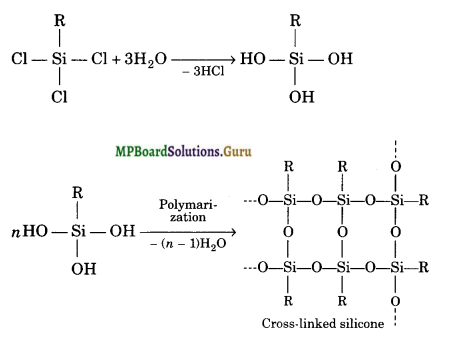### MP Board Class 11 Chemistry The p-Block Elements Important Questions and Answers

Question 1.
To which type of compounds, a name borane is given?
Hydrides of boron.

Question 2.
Which compound of boron is called inorganic benzene and why?
Borazine, it has structural similarity with benzene.

Question 3.
Which gas is produced when water is dropped over aluminium carbide?
CH4.

Question 4.
What is common oxidation state of group 13 elements?
+1 and +3.

Question 5.
What is the chemical formula of coloured compound formed during borax head test?
Metal metaborates MBO2, M'(BO2)2. (M = univalent, M’ = divalent)

Question 6.
Why BF3 acts as a Lewis acid?
It is an electron-deficient compound.

Question 7.
Arrange boron halides in the order of decreasing Lewis acid character.
BI3 > BBr3 > BCl3 > BF3.

Question 8.
What is state of hybridisation of carbon in (i) CO32-
(ii) HCO3-1 and
(iii) CO2?
(i) sp2
(ii) sp2
(iii) sp.

Question 9.
Name oxide of carbon that is neutral in character.
CO.Question 10.
What is dry ice and why it is so-called?
Solid CO2 is called dry ice. It is so-called because it sublimes in air.

Question 11.
What is buckminster fullerene?
C60 allotrope of carbon.

Question 12.
Why does elemental silicon not form a graphite-like structure as carbon does? Explain.
This is due to reluctance of silicon to form pπ-pπ multiple bonds because of large size of silicon atom. Hence silicon exists only in the diamond structure.

Question 13.
Anhydrous AlCl3 is covalent but hydrated AlCl3 is electrovalent. Why?
In the presence of H2O, Al2C16 dissociates into hydrated Al3+ and Cl ions due to high heat of hydration of these ions.

Question 14.
Why B-X bond distance in BX3 is shorter than theoretically expected value?
This is due to pπ-pπ back bonding of the fully filled p-orbital of halogen (X) into empty p-orbital of boron.

Question 15.
Why do boron halides form addition compounds with amines?
It is because BX3 have sextet of electrons and hence can accept a pair of electrons to complete its octet.

Question 16.
Which out of PbCl2 and PbCl4 can act as a good reducing agent?
Pb can exist in +2 and +4 oxidation state. As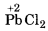can change to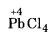, therefore it can act as a better reducing agent out of the two.

Question 17.
SiCl4 can be hydrolysed, why?
Due to presence of d-orbitals in its valence shell, it can extend its coordination number.

Question 18.
Why do boron halides tend to form addition compounds with ammonia or amines?
Being electron deficient, the boron compounds act as acceptor of electron pair from ammonia or amine molecules.Question 19.
Why is diborane referred to as electron-deficient compound?
Diborane is B2H6. The structure suggests 8 bonds are formed i.e., 8 × 2 = 16 electrons are required but electrons available for bending in diborane are 6 + 6 = 12. Hence, it is electron deficient.

Question 20.
Fluorine is more electronegative than chlorine, yet BF3 is less acidic than BCl3 Why?
It is because due to small size of fluorine the magnitude of interelectronic repulsions is more. As a result the tendency of back bonding is more and hence the acidic character will be less.

Question 21.
When metal X is treated with sodium hydroxide, a white precipitate (A) is obtained, which is soluble in excess of NaOH to give soluble complex (B).
Compound (A) is soluble in dilute HCl to form compound (C). The compound (A) when heated strongly gives (D), which is used to extract metals. Identify (X), (A), (B), (C) and (D). Write suitable equations to support their identities.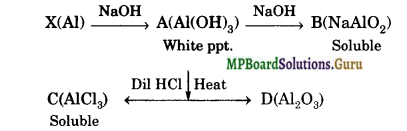Question 22.
State how will you separate COa and SO2 from their mixture?
Since the O.N. of C in CO2 is +4 which is the maximum for C, therefore, it cannot act as a reducing agent. In contrast the O.N. of S in S02 is also +4 but its maximum O.N. is +6, therefore, it can act as a reducing agent. This reducing gas can be removed by passing the mixture of these gases through acidified K2Cr2O7 solution.

Question 23.
SiO2 is solid but CO2 is a gas at room temperature. Why?
Carbon is able to form pπ-pπ bond with O-atom and constitute a stable non-polar molecule O=C=O. Due to weak interparticle forces its boiling point is low and it is a gas at room temperature. Si, on the other hand is not able to form pπ-pπ bond with O-atoms because of its relatively large size. In order to complete its octet, silicon is linked to four O-atoms around it by sigma bonds and thus it constitutes network structure which is responsible for its solid state.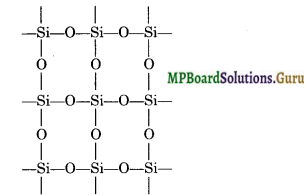Question 24.
SiCl4 can be easily hydrolysed but CCl4 does not hydrolyse. Why?
In SiCl4, the silicon atom has empty 3d orbitals in its valence shell which can accept an electron pair from H2O molecule resulting in co-ordinate bond. The co-ordinated molecule loses a molecule of HCl and as a result, a chlorine atom gets replaced by an OH group as shown.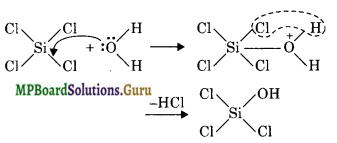In the same way, all the four Cl atoms are replaced by OH groups and the molecule gets completely hydrolysed. Carbon atom, on the other hand does not have vacant d-orbitals in 2nd level. Hence, it cannot accept electron pair form H2O molecule and therefore, CCl4 is not hydrolysed.

Question 25.
Boron nitride is called inorganic graphite. Why?
The structure of boron nitride (BN) is quite similar to that of graphite. In boron nitride B and N atoms are arranged in a network of flat regular hexagons similar to that in graphite. Thus C6 hexagons in graphite and (BN)3 hexagons in boron nitride are formed in the similar way. That is why boron nitride is called inorganic graphite.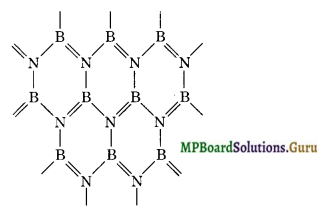Question 26.
Boron forms mainly covalent compounds.
B atom has there valence electrons. Due to extremely large value of ionisation enthalpy (IF1 + IF2 + IF3) it does not form ionic compounds readily and prefers to share its valedictions to form covalent bonds. Moreover, due to small size and high charge density, the B3+ ion has high polarising power, consequently, it tends to polarise the electron cloud of surrounding anions and acquire maximum covalent character.Question 27.
Why boron forms electron deficient compounds?
Boron has only three electrons in the outermost shell which it can share with other atoms. Hence in the compounds, there are only 6 electrons present around B-atom i. e., octet is not complete.

Question 28.
Aluminium chloride exists as a dimer, whereas boron does not, why?
Aluminium in AlCl3 has got six electrons in its valance shell. To complete its octet it requires two more electrons. To do so it accepts a lone pair of electrons from Cl atom of other neighbouring AlCl3.
In turn one of the chlorine atom of this molecule donates an electron pair to electron-deficient Al atom of neighbouring AlCl3 molecule. Hence a dimer is formed.
On the contrary boron due to its small size cannot arrange four large size chlorine atoms around it and hence exists as a monomer.

Question 29.
BBr3 is a stronger Lewis acid than BF3. Why?